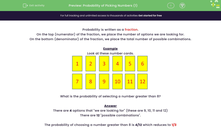# Find the Probability of Picking Certain Numbers

In this worksheet, students will calculate the probability of choosing or not choosing certain numbers.Key stage:  KS 3

Curriculum topic:   Probability

Curriculum subtopic:   Record, Describe and Analyse Probability Experiments

Popular topics:   Probability worksheets

Difficulty level:#### Worksheet Overview

Probability is written as a fraction.

On the top (numerator) of the fraction, we place the number of options we are looking for.

On the bottom (denominator) of the fraction, we place the total number of possible combinations.

Example

Look at these number cards.What is the probability of selecting a number greater than 8?

There are 4 options that we are looking for (these are 9, 10, 11 and 12)

There are 12 possible combinations.

The probability of choosing a number greater than 8 is 4/12 which reduces to 1/3

Remember that the fraction should always be reduced to its simplest form.

Are you ready to have a go at some questions?### What is EdPlace?

We're your National Curriculum aligned online education content provider helping each child succeed in English, maths and science from year 1 to GCSE. With an EdPlace account you’ll be able to track and measure progress, helping each child achieve their best. We build confidence and attainment by personalising each child’s learning at a level that suits them.

Get started